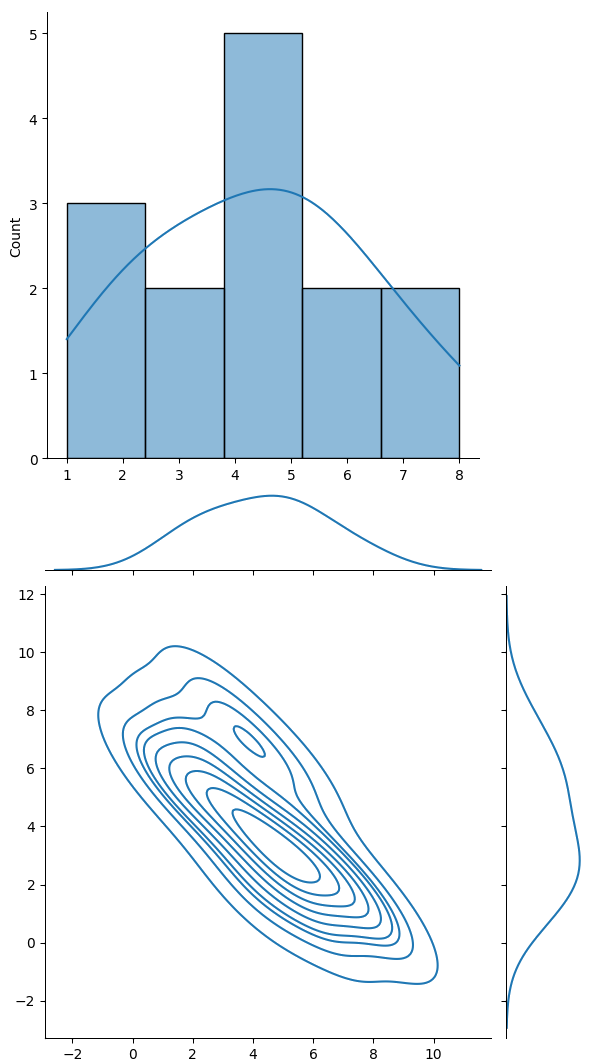Course Content

Data Science Interview Challenge

##Challenge 1: Visualizing Distributions

Understanding how data is distributed is fundamental in the data analysis process. Distributions help us to visualize the central tendencies, variability, and the presence of any outliers in our dataset. Seaborn, a statistical plotting library built on top of Matplotlib, provides a suite of tools that makes visualizing distributions a breeze.

The various plots and tools under Seaborn's distribution utilities can:

• Examine the distribution of a dataset.
• Visualize the relationship between multiple variables.
• Display the underlying probability distributions of datasets.

Using Seaborn to create distribution plots ensures that the viewer can get a comprehensive view of the data's distribution and its characteristics.Using Seaborn, visualize the distribution of a dataset:

1. Plot a univariate distribution of data using a histogram and overlay it with a kernel density estimate (KDE).
2. Visualize the bivariate distribution between two variables using a scatter plot and include a KDE plot to see the data's density.
Code Description
`sns.displot(x, kde=True)`

This function visualizes the distribution of the dataset `x`. The histogram represents the data's frequency, while the KDE (Kernel Density Estimate) plot gives an idea of the data's probability density.

`sns.jointplot(x=x, y=y, kind='kde')`

The `jointplot` displays a bivariate distribution between `x` and `y`. The scatter plot represents the individual data points, while the KDE on the margins and in the center shows the density of the data.

Everything was clear?

Section 5. Chapter 1